# 十大付费代理详细对比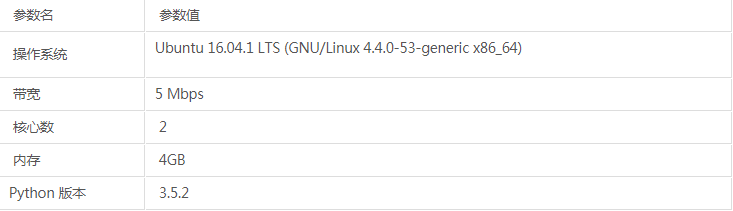```start_time = time.time()
requests.get(test_url, timeout=timeout, proxies=proxies)
end_time = time.time()
used_time = end_time - start_time```

```test_url = 'https://www.baidu.com/'
timeout = 60
def test_proxy(proxy):
try:
proxies = {
'https': 'http://' + proxy
}
start_time = time.time()
requests.get(test_url, timeout=timeout, proxies=proxies)
end_time = time.time()
used_time = end_time - start_time
print('Proxy Valid', 'Used Time:', used_time)
return True, used_time
except (ProxyError, ConnectTimeout, SSLError, ReadTimeout, ConnectionError):
print('Proxy Invalid:', proxy)
return False, None```

```max = 500
def main():
print('Testing')
used_time_list = []
valid_count = 0
total_count = 0
while True:
flag, result = get_page(api_url)
if flag:
proxy = result.strip()
if is_proxy(proxy):
total_count += 1
print('Testing proxy', proxy)
test_flag, test_result = test_proxy(proxy=proxy)
if test_flag:
valid_count += 1
used_time_list.append(test_result)
stats_result(used_time_list, valid_count, total_count)
time.sleep(wait)
if total_count == max:
break```

```import numpy as np
def stats_result(used_time_list, valid_count, total_count):
if not used_time_list or not total_count:
return
used_time_array = np.asarray(used_time_list, np.float32)
print('Total Count:', total_count,
'Valid Count:', valid_count,
'Valid Percent: %.2f%%' % (valid_count * 100.0 / total_count),
'Used Time Mean:', used_time_array.mean(),
'Used Time Var', used_time_array.var())```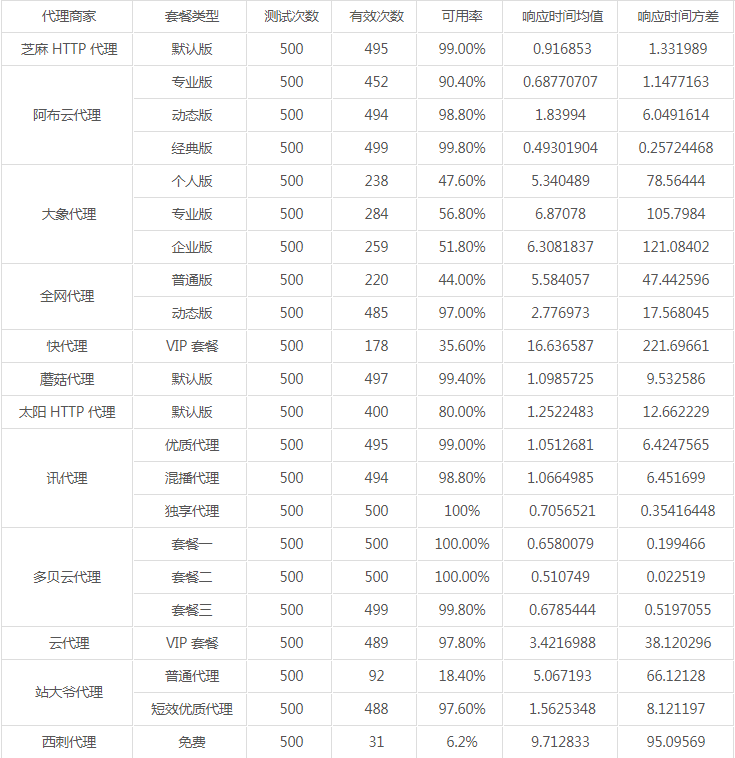·表中的响应时间方差越大，代表稳定性越低。

·阿布云代理经典版方差较小是因为它是长时间锁定了同一个 IP，因此极其稳定，但每秒最大请求默认 5 次。

·多贝云代理套餐一二方差较小是因为它是长时间锁定了同一个 IP，因此极其稳定，但每秒最大请求默认 20 次。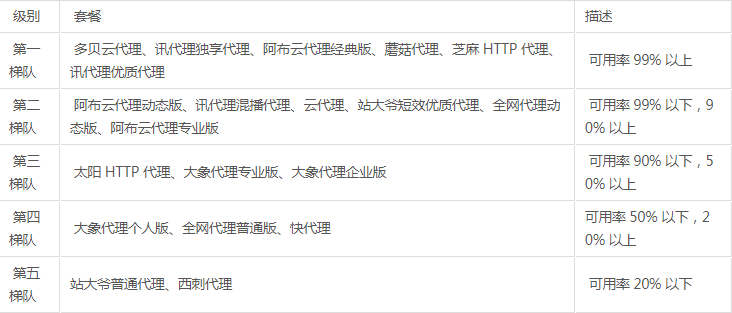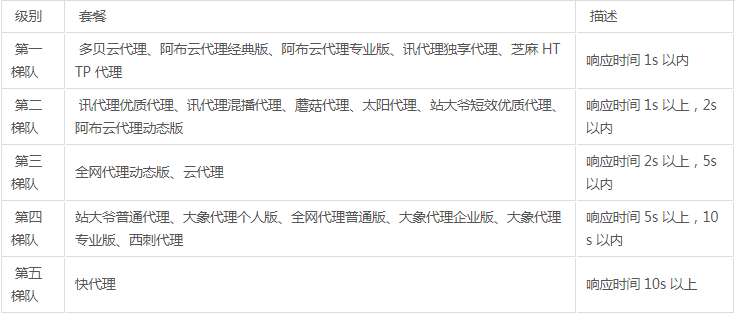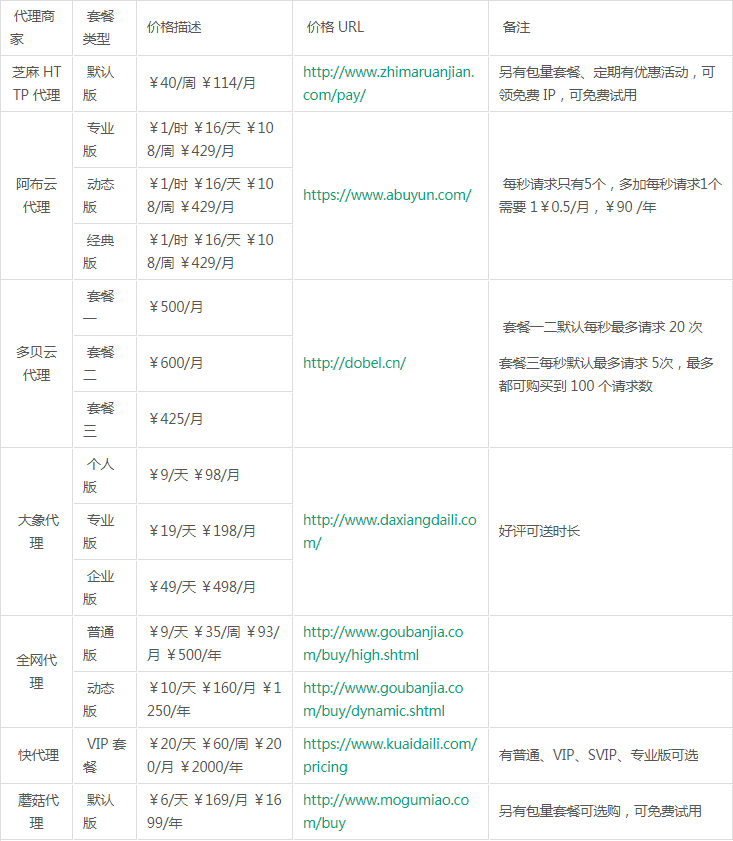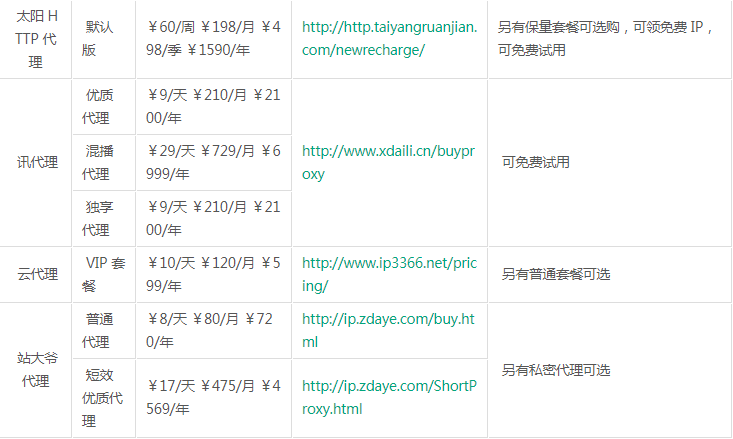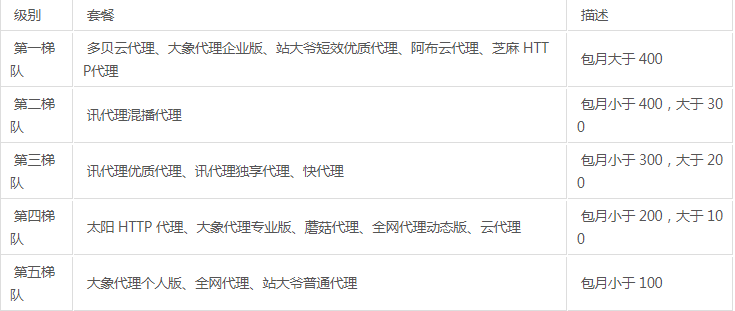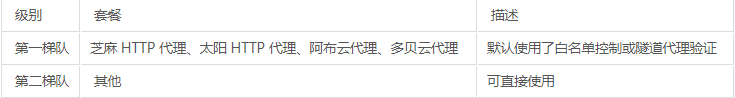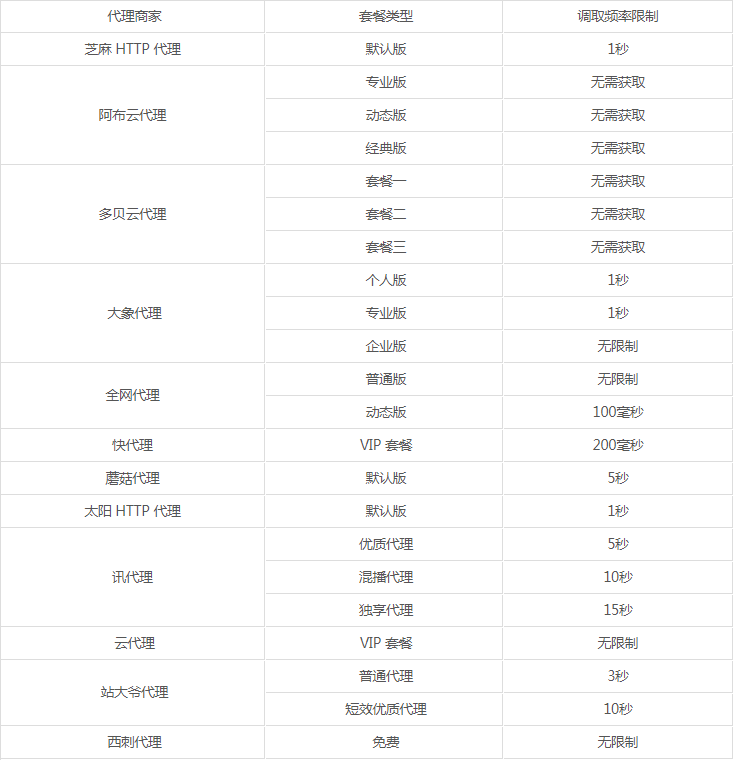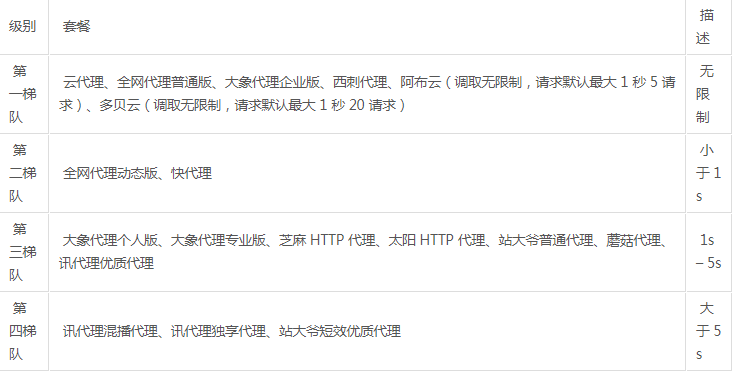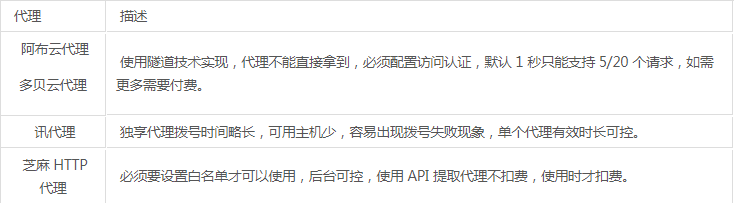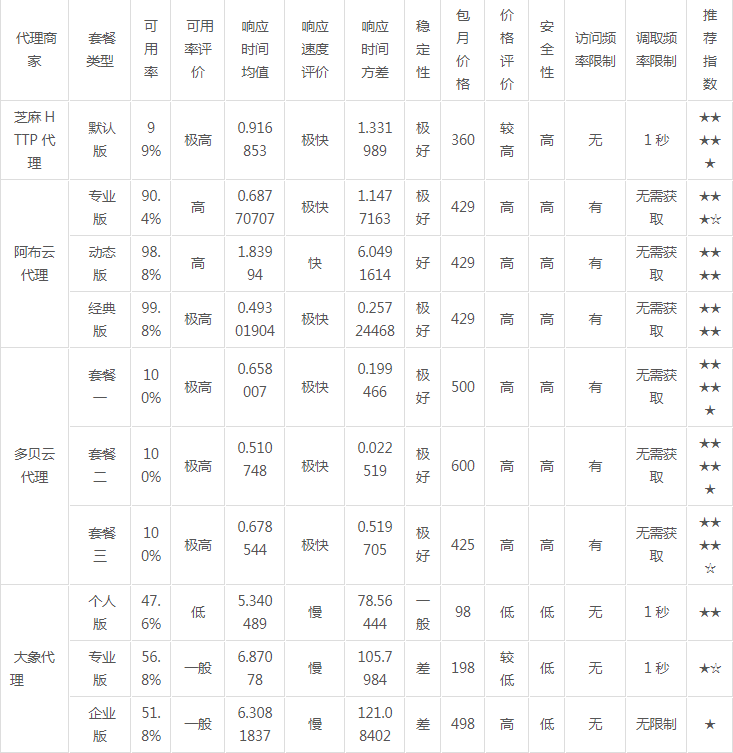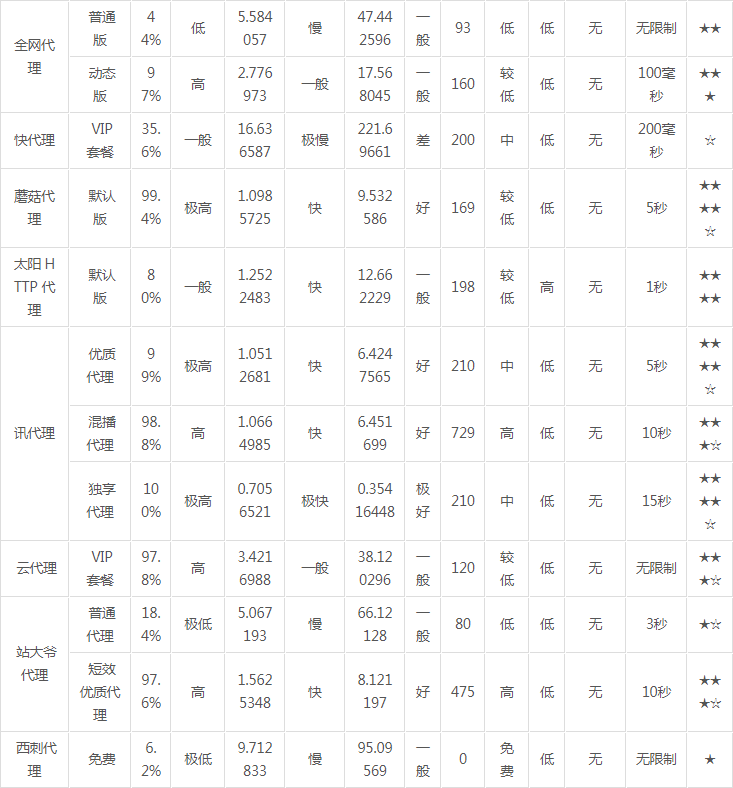Python开发开发项目